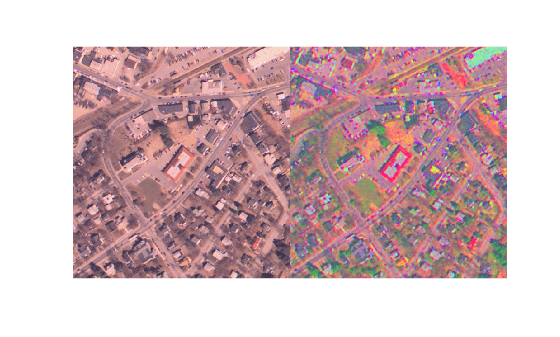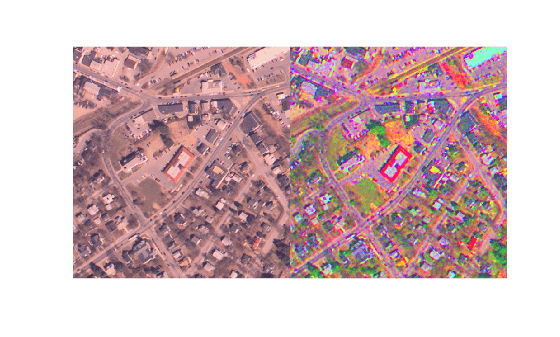# decorrstretch

Apply decorrelation stretch to multichannel image

## Syntax

``S = decorrstretch(A)``
``S = decorrstretch(A,Name=Value)``

## Description

example

````S = decorrstretch(A)` applies a decorrelation stretch to RGB or multispectral image `A` and returns the result in `S`. The mean and variance in each band of `S` are the same as in `A`. The primary purpose of decorrelation stretch is visual enhancement. Decorrelation stretching is a way to enhance the color differences in an image.```
````S = decorrstretch(A,Name=Value)` uses name-value arguments to control aspects of the decorrelation stretch, such as the target mean and standard deviation of each band.```

## Examples

collapse all

Read an image into the workspace.

`I = imread("westconcordaerial.png");`

Apply decorrelation stretching.

`S = decorrstretch(I);`

Display the original image and the enhanced image as a montage.

`montage({I,S})`Repeat the decorrelation stretching, and now apply linear contrast stretching using the `TargetSigma` name-value argument. Display the result.

```S2 = decorrstretch(I,TargetSigma=50); montage({I,S2})```## Input Arguments

collapse all

Image to be enhanced, specified as an RGB image or multispectral image of size m-by-n-by-`nBands`. For an RGB image, `nBands` is equal to 3.

Data Types: `single` | `double` | `int16` | `uint8` | `uint16`

### Name-Value Arguments

Specify optional pairs of arguments as `Name1=Value1,...,NameN=ValueN`, where `Name` is the argument name and `Value` is the corresponding value. Name-value arguments must appear after other arguments, but the order of the pairs does not matter.

Example: `S = decorrstretch(A,Mode="covariance")` specifies the decorrelation method as `"covariance"`.

Before R2021a, use commas to separate each name and value, and enclose `Name` in quotes.

Example: `S = decorrstretch(A,"Mode","covariance")` specifies the decorrelation method as `"covariance"`.

Decorrelation method, specified as of the following values.

• `"correlation"` — Uses the eigen decomposition of the band-to-band correlation matrix.

• `"covariance"` — Uses the eigen decomposition of the band-to-band covariance matrix.

Data Types: `char` | `string`

Target mean values of the output bands, specified as a numeric scalar or numeric vector of length `nBands`. By default, `TargetMean` is a 1-by-`nBands` vector containing the sample mean of each band, which preserves the band-wise means before and after the decorrelation stretch.

`TargetMean` must be of data type `double`, but uses the same values as the pixels in the input image. For example, if `A` is data type `uint8`, then `127.5` would be a reasonable value. If values need to be clamped to the standard range of the input or output image data types, it can impact the results.

Data Types: `double`

Target standard deviation values of the output bands, specified as a positive number or vector of positive numbers of length `nBands`. By default, `TargetSigma` is a 1-by-`nBands` vector containing the sample standard deviation of each band, which preserves the band-wise variance before and after the decorrelation stretch. The target standard deviation is ignored for uniform (zero-variance) bands.

`TargetSigma` must be of data type `double`, but uses the same values as the pixels in the input image. For example, if `A` is of data type `uint8`, then `50.0` would be a reasonable value.

Data Types: `double`

Linear contrast stretch following the decorrelation stretch, specified as a numeric scalar or 2-element numeric vector. Specifying a value of `Tol` overrides the value of `TargetMean` or `TargetSigma`. If you do not specify `Tol`, then by default `decorrstretch` does not perform linear contrast stretch.

`Tol` has the same meaning as in `stretchlim`, where `Tol = [LOW_FRACT HIGH_FRACT]` specifies the fraction of the image to saturate at low and high intensities. If you specify `Tol` as a scalar value, then `LOW_FRACT = Tol` and `HIGH_FRACT = 1 - Tol`, saturating equal fractions at low and high intensities.

Small adjustments to `Tol` can strongly affect the visual appearance of the output.

Data Types: `double`

Subset of `A` used to compute the band-means, covariance, and correlation, specified as a cell array containing two arrays of pixel subscripts `{rowsubs, colsubs}`. `rowsubs` and `colsubs` are vectors or matrices of matching size that contain row and column subscripts, respectively.

Use this option to reduce the amount of computation, to keep invalid or non-representative pixels from affecting the transformation, or both. For example, you can use `rowsubs` and `colsubs` to exclude areas of cloud cover. If not specified, `decorrstretch` uses all the pixels in `A`.

Data Types: `double`

## Output Arguments

collapse all

Decorrelation stretched image, returned as a numeric array of the same size and class as the input image, `A`.

## Tips

• The results of a straight decorrelation (without the contrast stretch option) may include values that fall outside the numerical range supported by the class `uint8` or `uint16` (negative values, or values exceeding `255` or `65535`, respectively). In these cases, `decorrstretch` clamps its output to the supported range.

• For class `double`, `decorrstretch` clamps the output only when you provide a value for `Tol`, specifying a linear contrast stretch followed by clamping to the interval `[0 1]`.

• The optional parameters do not interact, except that a linear stretch usually alters both the band-wise means and band-wise standard deviations. Thus, while you can specify `TargetMean` and `TargetSigma` along with `Tol`, their effects will be modified.

## Algorithms

A decorrelation stretch is a linear, pixel-wise operation in which the specific parameters depend on the values of actual and desired (target) image statistics. The vector `a` containing the value of a given pixel in each band of the input image `A` is transformed into the corresponding pixel `b` in output image `B` as follows:

`b = T * (a - m) + m_target`.

`a` and `b` are `nBands`-by-1 vectors, `T` is an `nBands`-by-`nBands` matrix, and `m` and `m_target` are `nBands`-by-1 vectors such that

• `m` contains the mean of each band in the image, or in a subset of image pixels that you specify

• `m_target` contains the desired output mean in each band. The default choice is `m_target = m`.

The linear transformation matrix `T` depends on the following:

• The band-to-band sample covariance of the image, or of a subset of the image that you specify (the same subset as used for `m`), represented by matrix `Cov`

• A desired output standard deviation in each band. This is conveniently represented by a diagonal matrix, `SIGMA_target`. The default choice is `SIGMA_target = SIGMA`, where `SIGMA` is the diagonal matrix containing the sample standard deviation of each band. `SIGMA` should be computed from the same pixels that were used for `m` and `Cov`, which means simply that:

`SIGMA(k,k) = sqrt(Cov(k,k), k = 1,..., nBands)`.

`Cov`, `SIGMA`, and `SIGMA_target` are `nBands`-by-`nBands`, as are the matrices `Corr`, `LAMBDA`, and `V`, defined below.

The first step in computing `T` is to perform an eigen-decomposition of either the covariance matrix `Cov` or the correlation matrix

`Corr = inv(SIGMA) * Cov * inv(SIGMA)`.

• In the correlation-based method, `Corr` is decomposed: `Corr = V LAMBDA V'`.

• In the covariance-based method, `Cov` is decomposed: `Cov = V LAMBDA V'`.

`LAMBDA` is a diagonal matrix of eigenvalues and `V` is the orthogonal matrix that transforms either `Corr` or `Cov` to `LAMBDA`.

The next step is to compute a stretch factor for each band, which is the inverse square root of the corresponding eigenvalue. It is convenient to define a diagonal matrix `S` containing the stretch factors, such that:

`S(k,k) = 1 / sqrt(LAMBDA(k,k))`.

Finally, matrix `T` is computed from either

`T = SIGMA_target V S V' inv(SIGMA) ` (correlation-based method)

or

`T = SIGMA_target V S V'` (covariance-based method).

The two methods yield identical results if the band variances are uniform.

Substituting `T` into the expression for `b`:

```b = m_target + SIGMA_target V S V' inv(SIGMA) * (a - m)```

or

`b = m_target + SIGMA_target V S V' * (a - m)`

and reading from right to left, you can see that the decorrelation stretch:

1. Removes a mean from each band

2. Normalizes each band by its standard deviation (correlation-based method only)

3. Rotates the bands into the eigenspace of `Corr` or `Cov`

4. Applies a stretch `S` in the eigenspace, leaving the image decorrelated and normalized in the eigenspace

5. Rotates back to the original band-space, where the bands remain decorrelated and normalized

6. Rescales each band according to `SIGMA_target`

7. Restores a mean in each band.

## Version History

Introduced before R2006a

expand all